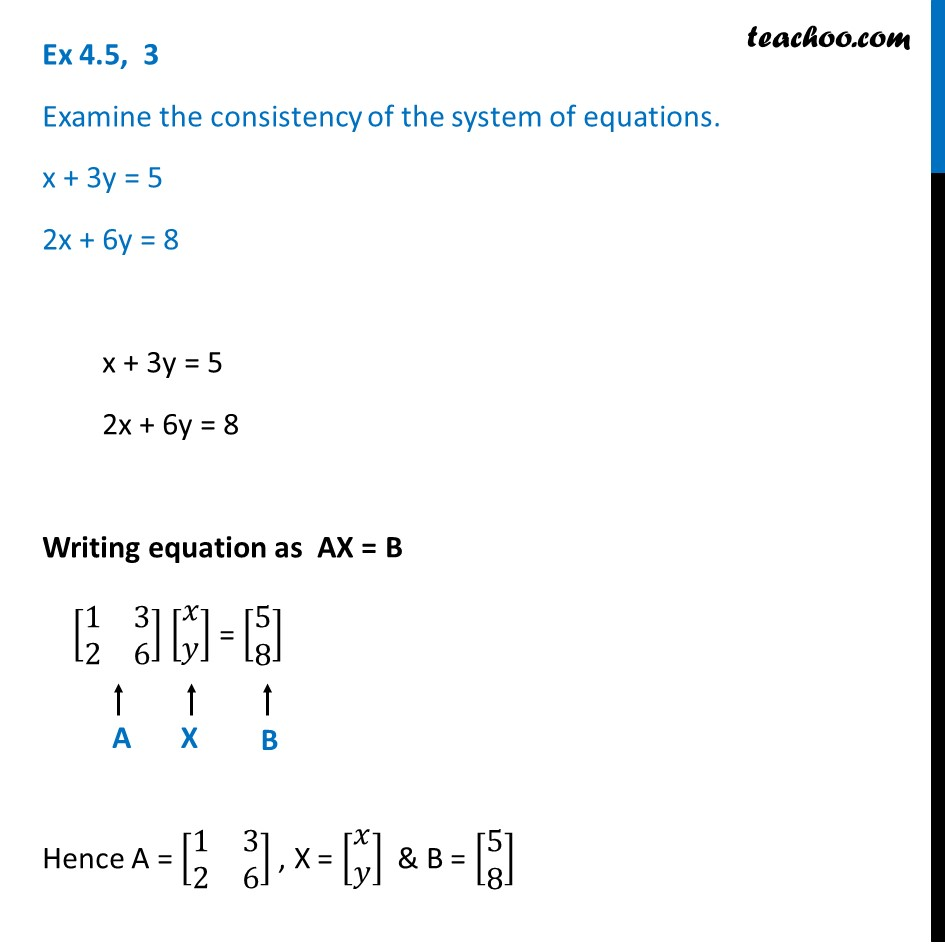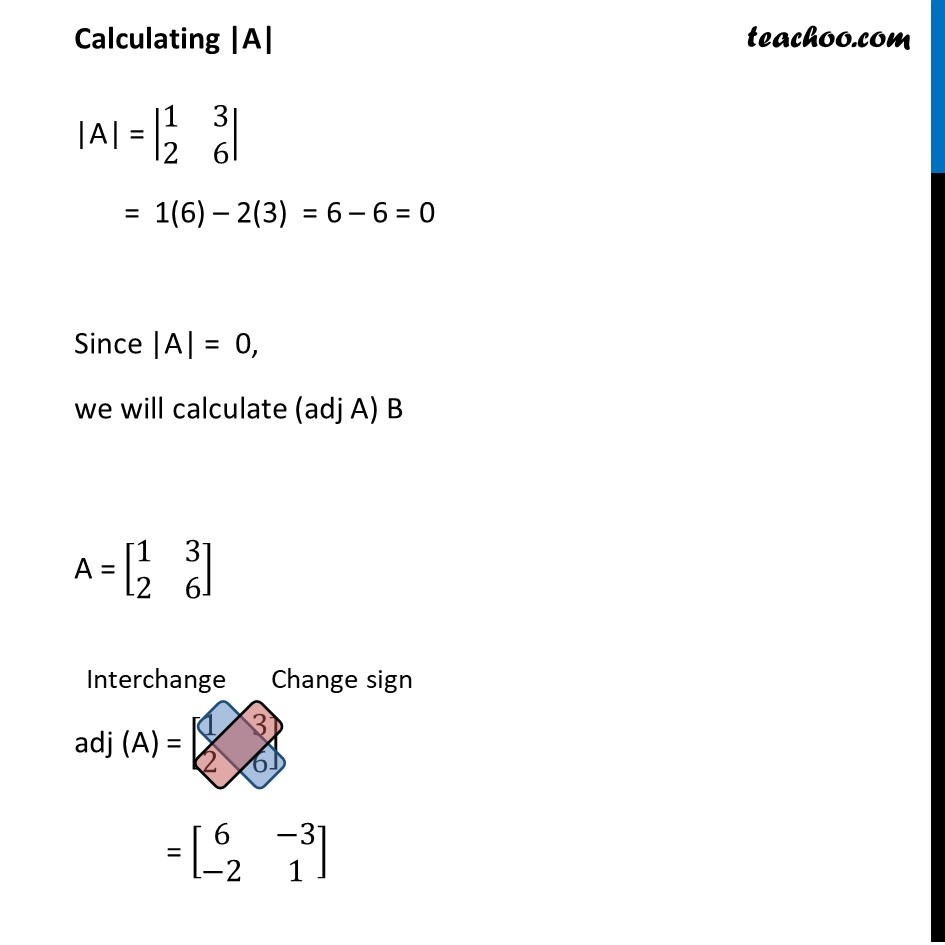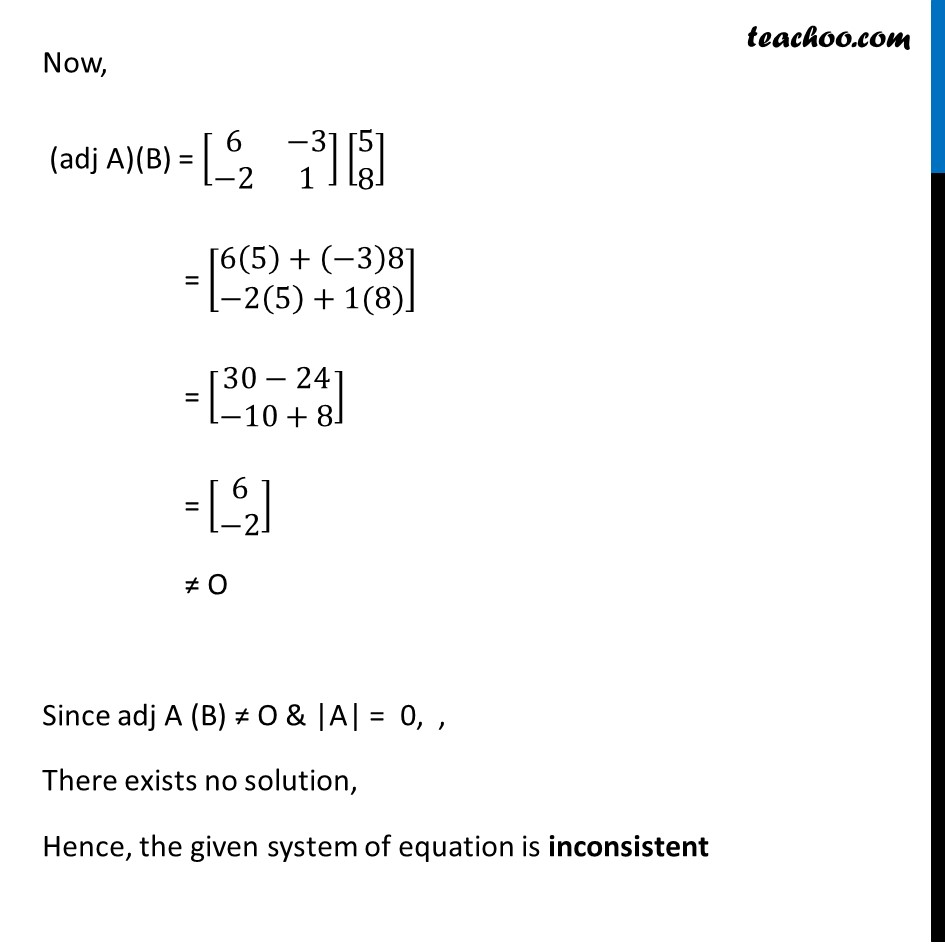Ex 4.5

Chapter 4 Class 12 Determinants
Serial order wiseLearn in your speed, with individual attention - Teachoo Maths 1-on-1 Class

### Transcript

Ex 4.5, 3 Examine the consistency of the system of equations. x + 3y = 5 2x + 6y = 8 x + 3y = 5 2x + 6y = 8 Writing equation as AX = B [■8(1&3@2&6)] [■8(𝑥@𝑦)] = [■8(5@8)] Hence A = [■8(1&3@2&6)] , X = [■8(𝑥@𝑦)] & B = [■8(5@8)] Calculating |A| |A| = |■8(1&3@2&6)| = 1(6) – 2(3) = 6 – 6 = 0 Since |A| = 0, we will calculate (adj A) B A = [■8(1&3@2&6)] adj (A) = [■8(1&3@2&6)] = [■8(6&−3@−2&1)] Now, (adj A)(B) = [■8(6&−3@−2&1)] [■8(5@8)] = [■8(6(5)+(⤶7−3)8@−2(5)+1(8))] = [■8(30−24@−10+8)] = [■8(6@−2)] ≠ O Since adj A (B) ≠ O & |A| = 0, , There exists no solution, Hence, the given system of equation is inconsistent